USArray
A Continental-scale Seismic ObservatoryFeatured Station

### Q24A

Divide, CO, USA# Sensors

A seismometer is a very sensitive instrument that can detect movements of Earth's surface. The surface is in constant motion due to natural and man-made phenomena. For example, the solid Earth tide is caused by the gravitational pull of the moon stretching Earth. Tucson slowly rises and falls twice a day about 40 cm due to the moon orbiting overhead. Mining explosions are a source of seismic energy. Ambient seismic noise amplitude is measured in nanometers, and can have a frequency bandwidth from 10s of Hz to days.

##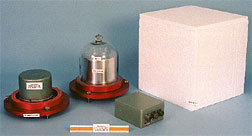• Flat to velocity 0.0028 - 10 Hz
• Dynamic range > 140 dB
• Requires extensive vault facilities
• 3-orthogonal components, 24-bit digitized at 40 Hz

##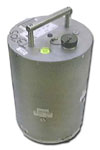• Flat to velocity 0.01 to 50 Hz
• Dynamic range > 140 dB
• Requires simple concrete pad with thermal insulation
• 3-orthogonal components, 24-bit digitized at 40 Hz

### Teledyne-Geotech KS54000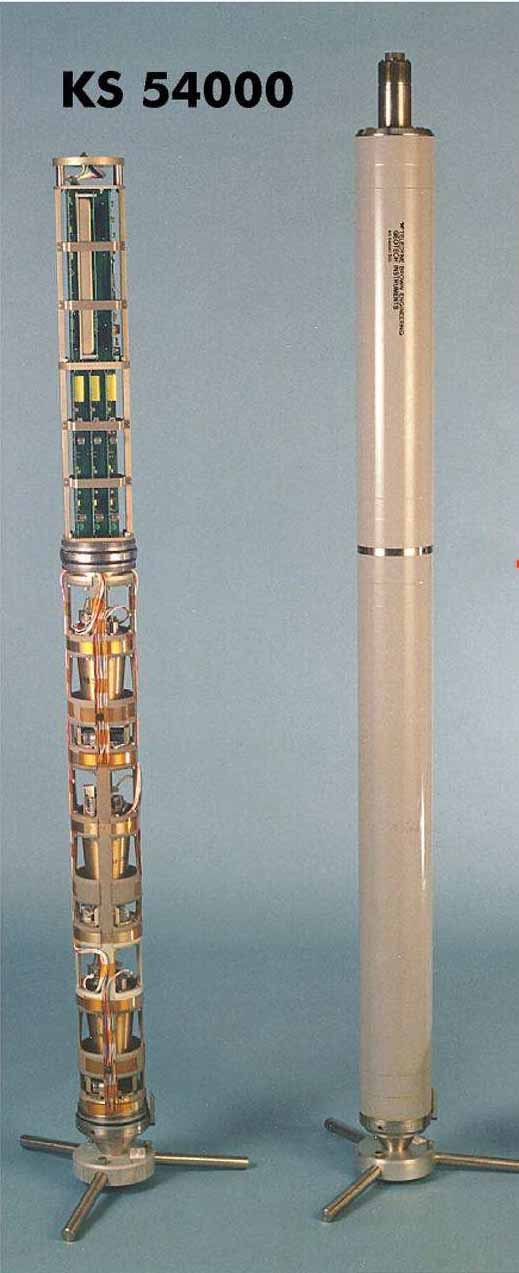• Flat to velocity 0.003 - 16 Hz
• Dynamic range > 140 dB
• Emplaced in 8" borehole, at a depth of 100m
• 3-orthogonal components, 24-bit digitized at 40 Hz

## Short-Period Seismometer

### Guralp CMG 40T-1Hz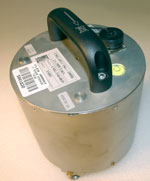• Flat to velocity 1s - 100 Hz response
• Dynamic range > 90 db
• 2000 V/m/s sensitivity
• Output 20 V peak-to-peak
• No mass locking required
• Power consumption = 38 Ma at 12 V DC
• Weight = 2.49 Kg
• Case diameter = 154 mm
• Case height = 207 mm
• Operating Temperature = -10 to + 75 deg C

## High-Frequency Seismometer

### Geospace GS11D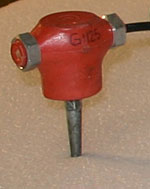• Natural Frequency 4.5 Hz
• Coil resistance = 380 Ohms
• Sensitivity = 0.32 V/cm/sec
• Damping with 380 Ohm coil = 762
• Moving mass = 23.6 g
• Height = 3.35 cm
• Diameter = 3.18 cm
• Weight = 111 g

## Strong-Motion Sensor

### Kinemetrics Episensor ES-T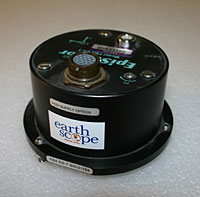• Dynamic Range > 155 Db
• Bandwidth DC to 200 Hz
• User selectable full-scale range +- 0.25 g, +-0.5 g, =+-1 g, +-2 g, or +-4 g
• Power consumption = 35 mA (Low Noise aAmp) @ 12 V DC
• Operating Temperature = -20 deg C to 70 deg C
• Physical size = 13.3 cm; diameter = 6.2 cm height